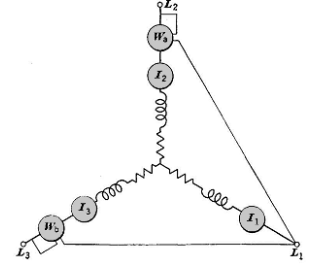# Three Phase Power Calculator

Three Phase Electric Power is basically a method of alternating current power generation, transmission, and distribution.It is the most common method used by elictrical grids to transfer power worldwide.Three-phase electricity is by its very nature a much smoother form of electricity than single-phase or two-phase power. It is this more consistent electrical power that allows machines to run more efficiently and last many years longer than their relative machines running on the other phases.Three-phase electric power systems have at least three conductors carrying alternating current voltages that are offset in time by one-third of the period.Formula: P = ( V12 * I2 * cos ( 30 + θ ) ) + ( V13 * I3 * cos ( 30 - θ ) )
Where, P = Three Phase Power,
V12 , V13 = Voltage,
I2,I3 = Current,
θ = Displacement Angle,
cos = Cosine

 Voltage V12 V Current I2 A Displacement Angle ° Voltage V13 V Current I3 A Three Phase Power W